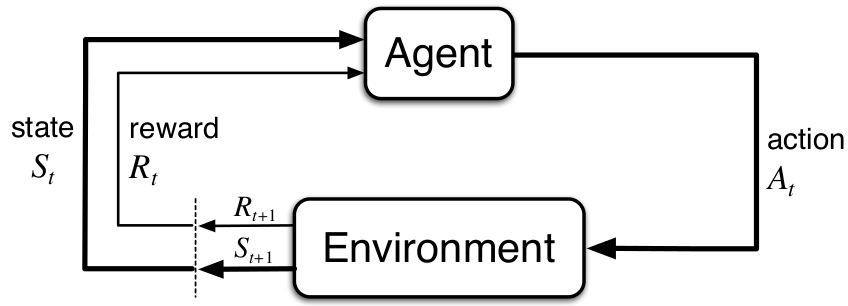# Objectives

• Brief introduction to Reinforcement Learning

# Introduction

As one of the main task in the project is to benchmark Deep Reinforcement Learning, with other approaches that are present in the Behavior Studio. In this post I will give a brief explanation about Reinforcement Learning .

## ConceptsFigure the book, Reinforcement Learning: An Introduction by Andrew Barto and Richard S. Sutton

Markov Decision Process framework models mathematically the Reinforcement Learning problem which comprehends the interaction between agent and environment  .

### Environment

The environment in this project is the whole track, here the agent will act using a camera.

### Agent

The agent would be the Formula 1 car, which will drive autonomously in the track following the red line.

### Actions

In the project actions are going to be considered deterministic, for example an action would be a medium throttle and 30 degrees to the left, if the action space is too large it could take longer to find the right policies (the best action for each state).

### State

Given that we are going to use a camera as perception, the states would be the images from the camera which could appended in succession to give a sense of recurrence.

### Reward

The reward signal $r$ is given by the environment after the agent has taken an action $a$ in a particular state $s$.

### Episode

An episode starts from an initial state $s_{0}$ until a terminal state which in our case would be when the Formula 1 car leaves the lane.

## Goals

The main goal of the reinforcement learning algorithms is to get the maximum discounted reward over an episode, also known as expected return denoted by $G_{t}$, the discount factor $\gamma, 0 \leq \gamma \leq 1$ controls the value of immediate rewards and long term rewards  .

$G_{t}=\sum_{t=0}^{\infty} \gamma^{t} r_{t}$

 Medium, Simple Reinforcement Learning: Q-learning

 OpenAI Spinning Up, Intro to RL OpenAI

 Deepmind, Playing Atari with Deep Reinforcement Learning

 B Ravi Kiran, et al. Deep RL for Autonomous Driving survey

 Andrew Barto and Richard S. Sutton, Reinforcement Learning: An introduction, MIT Press, 2018.